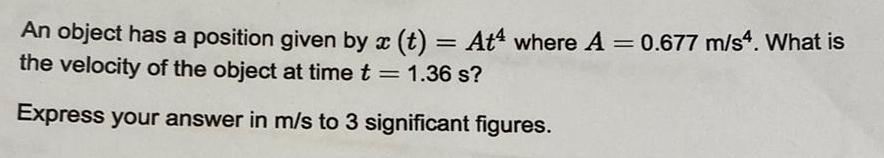Question:

# An object has a position given by x (t) = At where A = 0.677

Last updated: 8/9/2022An object has a position given by x (t) = At where A = 0.677 m/s4. What is the velocity of the object at time t = 1.36 s? Express your answer in m/s to 3 significant figures.